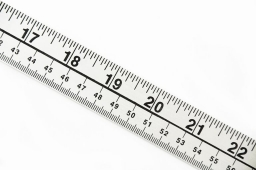# Increased 17813

The price of one meter of fabric increased by CZK 40, so three meters for the new fee were CZK 60 cheaper than four meters for the original price. What was the original, and what was the new cost of the substance?

a =  180
b =  220

### Step-by-step explanation:

b= 40+a
3b = 4a-60

b= 40+a
3·b = 4·a-60

a-b = -40
4a-3b = 60

Pivot: Row 1 ↔ Row 2
4a-3b = 60
a-b = -40

Row 2 - 1/4 · Row 1 → Row 2
4a-3b = 60
-0.25b = -55

b = -55/-0.25 = 220
a = 60+3b/4 = 60+3 · 220/4 = 180

a = 180
b = 220

Our linear equations calculator calculates it.Did you find an error or inaccuracy? Feel free to write us. Thank you!

Tips for related online calculators
Do you have a linear equation or system of equations and looking for its solution? Or do you have a quadratic equation?
Do you want to convert length units?